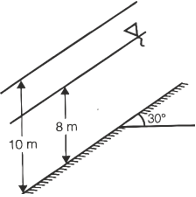Engineering Jobs   »   Civil Engineering Quiz

# GATE’22 CE:- Daily Practice Quiz 06-Sep-2021

IIT Kharagpur has released the GATE 2022 exam dates. As per the GATE 2022 exam date, the exam will be conducted on February 5, 6, 12 & 13. Candidates preparing for GATE 2022 must attempt this Civil Engineering Quiz to boost your GATE exam preparation.

Quiz: Civil Engineering
Exam: GATE
Topic: Miscellaneous

Each question carries 2 marks
Negative marking: 1/3 mark
Time: 12 Minutes

Q1. The dimension for kinematic viscosity is
(a) L/MT
(b) L/T^2
(c) L^2/T
(d) ML/T

Q2. In an iceberg, 15% of the volume projects above the sea surface. If the specific weight of sea water is 10.5 kN/m³, the specific weight of iceberg in kN/m³ is
(a) 12.52
(b) 9.83
(c) 8.93
(d) 6.83

Q3. A conventional flow duration curve is a plot between
(a) Flow and percentage time flow is exceeded
(b) Duration of flooding and ground level elevation
(c) Duration of water supply in a city and proportion of area receiving supply exceeding this duration
(d) Flow rate and duration of time taken to empty a reservoir at that flow rate

Q4. The factor of safety of an infinite soil slope shown in the figure having the properties c=0,ϕ=35°,y_dry=16 kN\/m^3 and y_sat=20 kN\/m^3 is approximately equal to(a) 0.70
(b) 0.80
(c) 1.00
(d) 1.20

Q5. A channel bed slope 0.0009 carries a discharge of 30 m³/s when the depth of flow is 1.0m. what is the discharge carried by an exactly similar channel at the same depth of flow if the slope is decreased to 0.0001?
(a) 10 m³/s
(b) 15 m³/s
(c) 60 m³/s
(d) 90 m³/s

Solutions

S1. Ans.(c)
Sol. SI unit of kinematic viscosity = m²/sec
∴ Dimension of kinematic viscosity = L^2/T

S2. Ans.(c)
Sol. Specific weight of ice-berg = (1-0.15) × 10.5
= 0.85 × 10.5
= 8.93 kN/m³

S3. Ans.(a)
Sol. A conventional flow duration curve is a plot between Flow and percentage time flow is exceeded.

S4. Ans.(a)
Sol. h=8m,Z=10m.
C=0
ϕ=35°
β=30°
y_d=16kN\/m^3
y_sat=20kN\/m^3
Assume:-y_w=10kN\/m^3
Factor of safety (F_s )
F_s=[1-(y_w h)/(y_sat Z)] Tanϕ/Tanβ
= [1-(10×8)/(20×10)] Tan35/Tan30
▭(F_s=0.73)

S5. Ans.(a)
Sol.
Q_1= 30 m³/sec
S_1 = 0.009
Q_2 = ?
S_2 = 0.0001
→ similar channel means that area and wetted perimeter are same.
Q_2/Q_1 =√(S_2/S_1 )
Q_2/30=√(0.0001/0.0009)
▭(Q_2=10 m^3 \/sec)

Sharing is caring!

Thank You, Your details have been submitted we will get back to you.